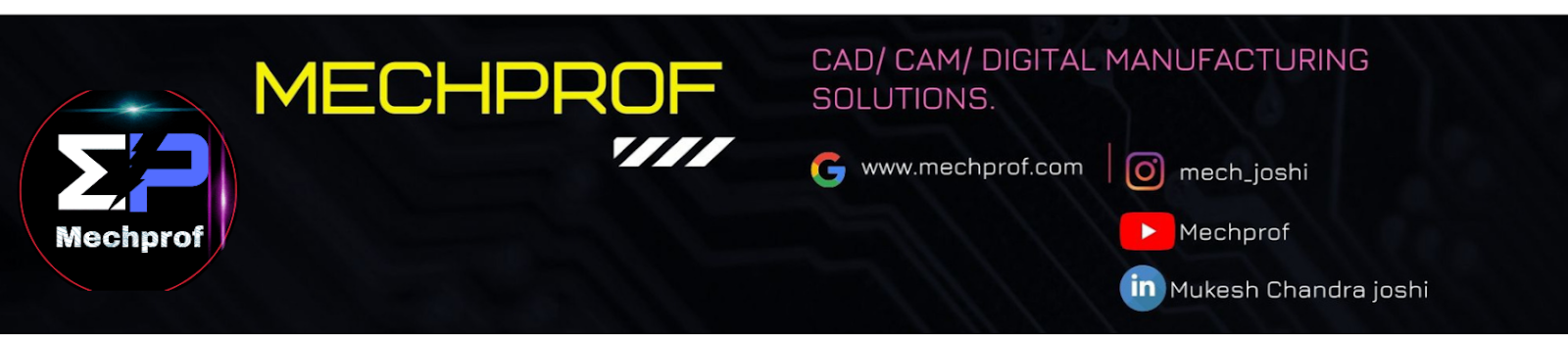Mechprof.com is The platform to learn Designing, Digital Manufacturing , manufacturing, simulation , CAM , CAD design, operation research, industrial engineering, mechanical tools, design, AutoCAD , Ansys, solidworks.

## Queuing Theory Part-2 :-

The two important parts of Queuing theory upon which the whole concept is based on.

(1) CUSTOMERS
(2) SERVICE PROVIDER

### Phases Of calculation :-

#### (a) Arrival O the customer :-

(1) Average / Expected or mean Arrival rate (入):- The rate at which customers are coming to get the services.

#### (b) Serving to a customer :-

(1) Average / Expected or mean service Rate ( μ ) :- The rate at which customers are served by the service provider known as Service Rate
Number of customers that are served within given time period known as Average Service rate.

(2) Utilization Rate (R) :- How much of the capacity is utilized is known as the utilization rate.

R = (入 / μ) = ( Average arrival rate / Average service rate ).

POSSIBILITIES :-

(a) If ,  入 > μ  :- (1) Infinite Queue
(2) Busy Service Provider
(3) Failure of service system.

(b) If ,  入 = μ  :- (1) Consumer comes & served
(2) No queue will be there
(3) Server will be busy.

(c) If , 入< μ  :- (1) No queue will be there
(2) There is Idle time for server.

(3) Probability of exactly "n" customers in the system :-

P(n) = R^n P(0)
P(n) = (入/μ)^n (1- 入/μ)

(4) Average number of customers in the system (Ls):-

Ls = (Utilization Rate / Idle Rate)
Ls =  (入/ μ- 入)

(5) Average number of customer in the system (Lq);-

Lq = (入^2 / μ(μ-入))

(6) Average waiting time for a customer in Queue (Wq) ;-

Wq = (Average numbers of customers in queue / Arrival Rate )
Wq = ( 入 / μ(μ-入) )

(7) Average waiting time for a customer in a system (Ws) :-

Ws = (Average number of customers in system / Average arrival rate)
Ws = (1/ μ-入)

(8) Expected Length of Non-Empty Queue :-

Lq' = (Expected number of customer in the queue / P( more than one customer in the system )
Lq' =  ( μ^2 / μ(μ-入) )

(9) Probability of waiting in queue :-

= e^ (-t/Ws)

or else
you can go to the

Thank you.